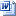## Display of grades and method of calculating the final grade for Test 1 – Evaluation of fundamental and specialized knowledge within the bachelor’s exam, July 2023 session

The results obtained in test 1 will be published on the website of the faculty at the address https://www.cs.ubbcluj.ro/anunturi/anunturi-licenta-si-disertatie/  and through the AcademicInfo platform, and the method of calculating the final grade in sample 1 it was the following:

Each subject was evaluated by two members of the evaluation committee. They awarded a full grade each, so that there was no difference of more than one point between the two grades. The grade of the subject is the arithmetic mean of the marks awarded. The scoring was done as follows:

Mathematics field

1. Specialization Mathematics: the arithmetic mean of the grades obtained in the three Mathematics subjects (Algebra, Mathematical Analysis, Geometry);
2. Specialization Mathematics and Computer Science: 2/3 grade in Mathematics + 1/3 grade in Computer Science. The grade from the Mathematics subject was calculated as the arithmetic mean of the grades obtained in the three Mathematics subjects (Algebra, Mathematical Analysis, Geometry);

Informatics field

1. Specialization Computer Science: the arithmetic mean of the grades obtained in the three subjects of Informatics (Algorithmic and programming, Databases, Operating systems).

SUBMISSION OF APPEALS

Appeals to test 1 can be submitted by graduates on July 4, 2023 between 9:00 a.m. and 2:00 p.m. at the address:  https://forms.microsoft.com/e/eULw2pCKN92023 bachelor’s appeal form